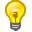# Language Complexity, Part 1

A simple view of languages

How complex is the English language? The question has many dimensions and nuances. What does complexity mean, and how could it be measured? This a tough nut to crack, so in this post we’ll make a retrenchment and reconsider the question in a more formal setting — computer science.

In this setting, a language gets identified with its extension — the collection of all its sentences. So English gets modeled as just a large repository of sentences. Although this is an ‘impoverished’ view of language, it still has value, as simpler models can still give us ideas to work with.

It is easy to see that the extension of the English language is an infinite set of sentences, as it includes for example:

• The father of Adam did not exist.
• The father of the father of Adam did not exist.
• The father of the father of the father of Adam did not exist.

One can also consider very small languages. For example, the sublanguage of English consisting of the sentences where the subject and object are either Alice or Bob, and the only verb is ‘likes’:

• Alice likes Alice.
• Alice likes Bob.
• Bob likes Alice.
• Bob likes Bob.

Let’s call this language AB:

AB = {“Alice likes Alice”, “Alice likes Bob”, “Bob likes Alice”, “Bob likes Bob”}.

Here, a ‘sentence’ means something rather simple – a string of characters that belongs to the language. So the sentence “Bob likes Bob” just means [B, o, b, SPACE, l, i, k, e, s, SPACE, B, o, b]. And the word “Alice” is just a shorthand for the string [A, l, i, c, e]. There are no semantics here.

Here are a couple of other examples of formal languages.

• The set of all strings involving just the characters 0 and 1, which start with 0 and alternate. That is: {“0”, “01”, “010”, “0101”, …}. This is a simple example of an infinite language.
• The set of prime numbers, written out as strings: [“2”, “3”, “5”, “7”, “11”, “13”, “17”, …]. Another infinite language.

Now that we know what these ‘languages’ are, we are ready to talk about how to measure their complexity.

This is a custom html block Here is an image: